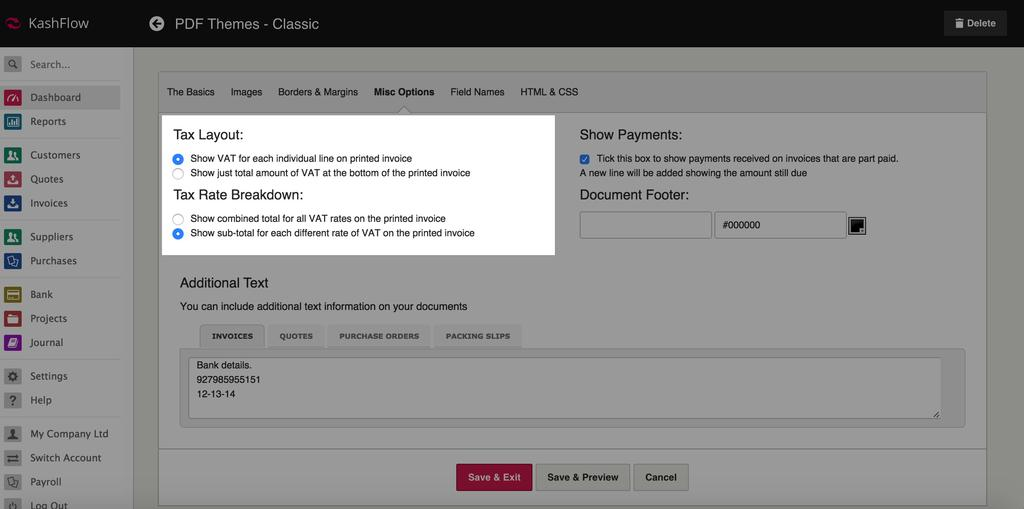# VAT Calculation Methods

KashFlow can calculate the VAT on your line items in two ways – either by line total or by calculating on individual unit price. Both methods are recognised as correct by HMRC, but making sure you’ve chosen the right method of calculating VAT is really key. Choosing a method and sticking with it is important because accounting packages (including KashFlow) typically round to two decimal places and having the wrong option ticked can occasionally result in rounding differences if you sell items for a fixed price including VAT.

You can choose the correct VAT calculation formula by going to Settings > PDF themes > Choose your theme > Misc Option > Tax Option > Calculation Method.

Please note: If you have raised an invoice calculated under the wrong method you will need to either delete the line item and put it back in, or manually re-calculate the VAT on it – otherwise the VAT amount will not be updated.

## Calculate tax on line total

This will multiply the quantity by the net rate before applying the tax. For example;

Product costing £3.43 excluding VAT and total sold is 33 at 20% VAT:

£113.19 (£3.43 x 33) + £22.64 (22.638 rounded to nearest penny, VAT at 20%) = £135.83

## Calculate tax on individual unit price

This will add the VAT rate to the individual unit price of the item, and then multiply the gross by the quantity.

Product costing £3.43 excluding VAT and total sold is 33 at 20% VAT:

£3.43 + £0.69 (0.686 rounded to nearest penny, VAT at 20%) x 33 = £135.96If you’ve changed your VAT calculation formula be sure to click the Update button at the bottom of the page, or the change won’t be registered and, as mentioned above, don’t keep switching between the two methods or you may encounter problems with rounded numbers not matching!